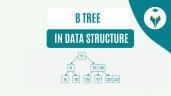Cross Join in SQL

# Cross Join in SQL

clickHere
Updated on Mar 31, 2023 16:41 IST

SQL Cross Join combines each row of one table with each row of another table and the number of rows in the result set is the product of number of rows in both the tables. In this article, we will briefly discuss how to combine two or more tables using SQL Cross Join.

In the previous articles, we have discussed different types of Joins in SQL, such as Inner JoinLeft Join, and Right Join. This article will discuss another type of SQL Join, i.e., Cross Join.

Must Check: SQL tutorial

## What is SQL Cross Join?

Similar to other Joins in SQL, cross join is used to combine two or more different tables. Cross join in SQL combines each row of one table with each row of another table and returns the cartesian product of all the table elements.

In simple terms, cross join in SQL returns all the possible combinations of both tables.

• The result set of Cross Join is given in the ordered pair in which the first element is from table1 and the second element is from table 2.
• Number of rows in the result set is equal to the product of the number of rows in the first table and the number of rows in the second table.

Read Also: Update Query in SQL

The product of cross join can be easily understood by the given below example:The above picture contains the two sets, each containing three elements, and when the cross join (cartesian product) is performed, the result set will contain nine elements.SELECT Statement in SQL
This article will help you understand some of the most common SQL SELECT use cases with examples. Large amounts of data in diverse types are stored in databases. Have you...read moreIntroduction to SQL
This article will teach you basic and advanced concepts of SQL. It concepts, such as what is a Database Management System, what is SQL, SQL Data types, SQL Statements, and...read moreHow to Create, Update, Insert and Delete SQL Views?
This article will give you a detailed insight on SQL views. You will learn the method to create, update, insert, drop and delete SQL views.

Let’s take an example to get a better understanding of how SQL cross works:

Example: Let there be two tables, table 1 ( containing student name) and table 2 ( containing subject name), and when we cross join both the tables, we will get the following result.Syntax

The query of Cross Join can be written in two ways:

1st Method:

` `
`SELECT column_namesFROM table_1    CROSS JOIN table_2;Copy code`

2nd Method:

` `
`SELECT column_namesFROM table_1, table_2;Copy code`

Note:

• Both the methods will produce the same result
• Unlike the Inner Join, Left Join, and Right Join, SQL Cross Join does not require any joining conditions.Introduction to Normalization – SQL Tutorial
This article will cover what Normalization in SQL is, its forms, such as 1NF, 2NF, etc., and examples.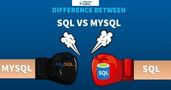What is the Difference Between SQL and MySQL?
SQL is query programming language to manage RDBMS while MySQL is RDBMS that uses SQL.Difference between SQL and NoSQL
This article designed for beginners will teach you difference between SQL and NoSQL. The tutorial includes concepts, such as what is a SQL, what is NoSQL, and more.

Now, let’s use the same above example and write the query to produce the result:

Firstly we will create both Student and Subject tables:

` `
```CREATE TABLE Student (    Id int PRIMARY KEY    Student Name VARCHAR (56)); INSERT INTO Student VALUES (1, Kartik);INSERT INTO Student VALUES (2, Amit);INSERT INTO Student VALUES (2, Shewtank);  SELECT *FROM Student;Copy code```

The above query will produce the following table:

` `
```CREATE TABLE Subject (    Id int PRIMARY KEY    Subject Name VARCHAR (56)); INSERT INTO Subject VALUES (1, English);INSERT INTO Subject VALUES (2, Hindi);INSERT INTO Subject VALUES (2, Math);      SELECT *FROM Subject;Copy code```

The above query will produce the following result:

Now, finally, we will write the query to combine both the table using cross join:

` `
`SELECT st.Student Name, su.Subject NameFROM Student stCROSS JOIN Subject su;Copy code`

The above query will produce the following table:

We were looking for this; the cross join query (the result set) returns the same nine entries.
Till now, we have learned how to join two tables using cross join, and now we will see how to join more than two tables using SQL Cross Join.

## SQL CROSS JOIN with Three Different Tables

Let there are three different tables Menu, Restaurant Name, and Location

Restaurant Name:

Location

Now, we want to see all the possible combinations where these food items are available. The below query will help you to find all the possible combinations:

` `
```SELECT      m.Food Item AS Item,     r.Restaurant Name,     l.Location FROM Menu mCROSS JOIN Restaurant rCROSS JOIN Location l;Copy code```

Output

## Conclusion

In this article, we have briefly discussed how to join two tables in sql using cross join with examples.

Keep Learning!!

Keep Sharing!!

clickHere

Vikram has a Postgraduate degree in Applied Mathematics, with a keen interest in Data Science and Machine Learning. He has experience of 2+ years in content creation in Mathematics, Statistics, Data Science, and Mac... Read Full Bio

## Trending Management CoursesCoursera4.7Data Analytics
Great Learning4.2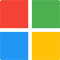Microsoft Certified: Data Analyst Associate
Microsoft4.9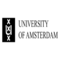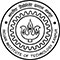## Top Picks & New Arrivals